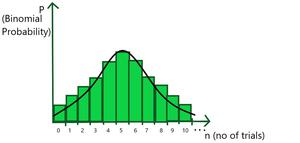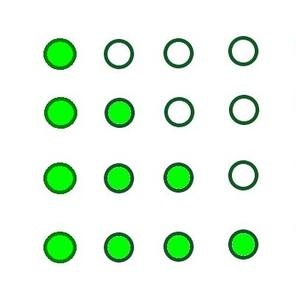GFG App
Open AppBrowser
Continue

# What is Binomial Probability Distribution with example?

Probability is the likelihood of something happening. When we say the probability of something, it means how likely that something is. Some events have a high probability and are very likely to happen, and some have less probability which means they are very unlikely to happen. Examples – The probability of getting a tail on tossing an unbiased coin is 1/2 and the probability of getting a number greater than 4 on rolling dice is 1/3. In other words, it is the measure of the chance that the event will occur as a result of an experiment.

Probability theory is a very powerful instrument for organizing, interpreting, and applying information which is very useful in various domains like data science, trading, betting of horses, etc.

### Binomial Experiment

A Binomial experiment is an experiment in which there are a fixed number of trials (say n), every trial is independent of the others, only 2 outcomes: success or failure, and the probability of each outcome remains constant for trial to trial. Examples of the binomial experiments,

### Binomial Probability

When there is given any binomial experiment in which we are performing random experiments multiple times (for example, tossing a coin 7 times or rolling a dice 10 times ), then finding out the probability of a certain outcome in n trials is called its binomial probability. Consider a binary experiment has n independent trials with two outcomes:

• Success
• Failure

Now the Probability of getting r successes in n trials is:

P = nCr.pr.qn-r

where p = probability of success and q = probability of failure such that p + q = 1.

Graphical Representation of symmetric Binomial Distribution

The binomial distribution consists of multiple Bernoulli’s events. The difference between Bernoulli’s distribution and Binomial distribution is that the expected value of Bernoulli’s distribution gives the expected outcome for a single trial while the expected value of Binomial distribution suggests the number of times expected to get a specific outcome. The graph of binomial distribution represents the likelihood of attaining our desired outcome a specific number of times.### Sample Problems

Question 1: If an unbiased coin is tossed 7 times, then find out the probability of getting exactly 3 heads.

Solution:

Given number of trials(n) = 7, number of success(r)= 3

= Probability of success = Probability of getting a head in a trial (p) = 1/2

= Probability of failure = Probability of not getting a head in a trial (q) = 1/2

Now applying binomial probability formula:

= Probability of getting exactly 3 heads (P) = nCr.pr.qn – r

= 7C3.(1/2)3 × (1/2)4

= (7!/3!.4!) × (1/2)7

= 35/128

Question 2: A dice is thrown 5 times, and getting an odd prime number is considered a success. Find the probability of:

1. Exactly one success
2. At least one success

Solution :

Given:  Number of trials (n) = 5,

Number of odd prime numbers from 1 to 6 = 2 (3, 5)

= Probability of success = Probability of getting a 3 or 5 on the dice(p) = 2/6 = 1/3

= Probability of failure = Probability of not getting 1, 2, 4, 6 on the dice(q) = 1 – 1/3 = 2/3

• Exactly one success(r = 1)

Applying binomial probability formula:

=> Probability of getting exactly 1 success (P) = nCr.pr.qn-r

= 5C1. (1/3)1. (2/3)4

= 80/243 (Ans)

• At least one success( r = 1, 2, 3, 4, 5)

Now since it is given at least one succes, add all the binomial probabilities for r = 1, 2, 3, 4, 5

Applying binomial probability formula:

= Probability of getting at least 1 success (P) = P(r = 1) + P(r = 2) + P(r = 3) + P(r = 4) + P(r = 5)

(getting 1 success) (2 success) (3 success) (4 success) (5 success)

= 1 – P(r = 0) (getting no success)

= 1 – nC0.p0.qn – 0

= 1 – 5C0.(1/3)0.(2/3)5

= 1 – 32/243

= 211/243

Question 3: Joker draws 4 cards from a well-shuffled deck of 52 cards with replacement. Find the probability that he draws at least 3 kings from the deck.

Solution:

Given: Number of cards to be drawn(n) = 4

Success =  getting a king

Probability of getting a king card from 52 random cards(p) = 4/52 = 1/13 (Since total no of kings = 4 and each card is replaced after every pick)

Probability of failure(q) = 1 – 1/13 = 12/13

= Probability of getting at least 3 king in this case = P(r = 3) + P(r = 4)

(getting 3 kings) (getting 4 kings)

Applying binomial probability formula = 4C3.(1/13)3.(12/13)1 + 4C4.(1/13)4.(12/13)0

= (1/13)3.(4.12/13 + 1/13) (Taking common on both sides)

= 49/28561

Question 4: You are giving an MCQ test having only 5 questions. In each question, 4 options are given and each question can have one or more than one correct answer. Since you have not studied anything for the test, you decide to mark all the answers at random. Find the probability that you get exactly 3 questions correct out of 5, to just pass your examination.

Solution:Given: Number of questions(n) = 5

Now to find the probability of success, first, find the total

no of the ways a question can be answered.

As shown in the figure above, there are 4 cases :

= number of ways to answer a question when only 1 option is correct = 4C1 = 4 ways

= number of ways to answer a question when 2 options are correct = 4C2 = 6 ways

= number of ways to answer a question when 3 options are correct = 4C3 = 4 ways

= number of ways to answer a question when 4 options are correct = 4C4 = 1 way

Adding up all ways, the total no of ways = 15 ways.

Now out of these 15 ways, only one will be correct for a particular question.

Thus, the probability of success(i.e. getting a correct answer) = 1/15

And the probability of failure = 1 – 1/15 = 14/15

Now,

The probability that you get exactly 3 question correct out of 5

= 5C3.(1/15)3.(14/15)

= 10. (196/759375)

= 392/151875

My Personal Notes arrow_drop_up